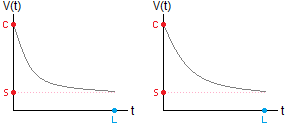# How to Calculate Double Declining Balance Depreciation

Double Declining Balance Calculator
double decline             150% declinePurchase Price \$
Scrap Value \$
Lifespan      years

Depreciation models are mathematical formulas that give you the value of an asset after a certain number of years. Business owners use asset depreciation to calculate their losses and to appraise the total value of their holdings.

The double declining balance method of depreciation assumes that the asset decreases by a fixed percentage rate each year. It is similar to exponential depreciation except for two small differences. (1) The percent decrease each year depends only on the useful life of the object. (2) At some point the depreciation model switches to straight line depreciation, either because the asset has reached its salvage value, or because the straight line model shows more depreciation. Because of (2), you must run the double declining balance method alongside the straight line method, and compare them.

### Formula for Double Declining Balance Depreciation

If the useful life of the object is L years, then assume that the annual percent decrease in the object's value is 2/L. If the initial cost of the item is C, then the equation for the asset's value after t years is

V(t) = C(1 - 2/L)t.

This equation is valid for all values of t up until the point where the asset attains its scrap value, or the straight line depreciation model actually shows a lower value. Here is an example to illustrate how to use double declining depreciation.

Example: Suppose a printer costs \$6000, its estimated useful lifespan is 10 years, and its scrap value is \$100. Since 2/10 = 0.2, the printer decreases in value by 20% each year, and the value equation is V(t) = 6000(0.8)t.

 Year End of Year ValueV(t) = 6000(0.8)t (using DDB) End of Year ValueV(t) = 6000 - 590t (using SL) 1 \$4800 \$5410 2 \$3840 \$4820 3 \$3072 \$4230 4 \$2458 \$3640 5 \$1966 \$3050 6 \$1573 \$2460 7 \$1258 \$1870 8 \$1007 \$1280 9 \$805 \$690 10 \$644 \$100

The DDB method gives a lower value up until year 9, at which point the SL method yields a lower value. Thus, for years 9 and 10, you switch to the straight line depreciation method to figure the asset's diminished value.

### Variation: 150% Declining Balance

The 150% declining balance is the same as double declining balance, except that you use 1.5/L instead of 2/L. Using the example of a printer with a lifespan of 10 years, the equation for its value is

V(t) = 6000(0.85)t.

After 7 years, the model switches to straight line depreciation.